# Simple Regression using Deep Neural NetworkPhoto by Markus Spiske on Unsplash

There are plenty of complex neural network examples out there to explore, but it is always better to start from the basics as it gives you more insights on the things working on rudimentary levels. So in this article, I will be using a simple deep neural network approach to predict a regression problem, which learns to predict a sine wave using the noisy signal. Moreover, there are many different methods to address this problem but I am going to implement this using TensorFlow. And this article focuses on the programming part and not the mathematics behind it.

# Mathematical Description

As described earlier, we are interested in modelling a function which predicts sine wave from a noisy sine wave. y=f(x;θ)+𝞊 where y is the desired random output vector with certain dimension M, and x is the input feature vector with dimension N. θ is the unknown parameter which is used to predict the output y. 𝞊 is a noisy parameter which is considered to be from the known probability distribution.

There is the loss function l(x,y;θ) which give the loss value for each pair of (x, y), then the cost function is calculated, which is an average loss of overall training set given by L(θ) = ⅀ l(x(n),y(n);θ) /N.

## Steps involved in training:

1. Defining constants and dataset segmentation
2. Creating hidden layers in the network
3. Training and testing the network

Well, there may be many other steps while training the neural network but it is application-specific in some case.

# Defining constants and dataset segmentation

These are some constants that are used in the code, which is declared globally. Data segmentation and processing involve fetching the data or dataset and processing it to feed it to the neural network. But in our case, we generate our own that is a simple sine wave as Observation and Ground truth data. Next, we divide this data into three parts namely train data, test data and validation data. As the name suggests, training data is used to train the network with the corresponding ground truth. Test data is used to check the generalization or unbiased evaluation to decide as to how good the model predicts to new unseen data. Validation data is used to fine-tune the hyperparameters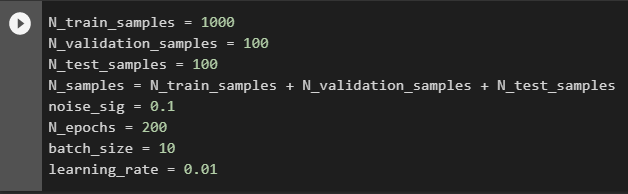Constants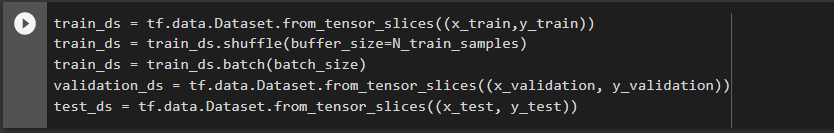Data Segmentation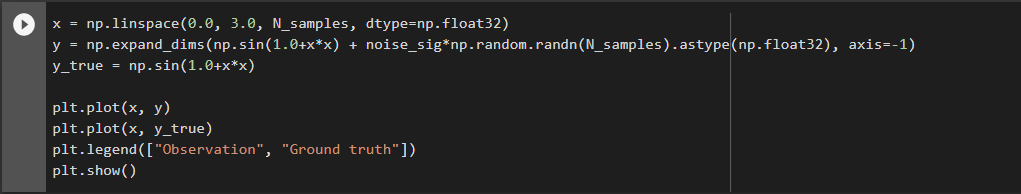Visualize the observation and ground truth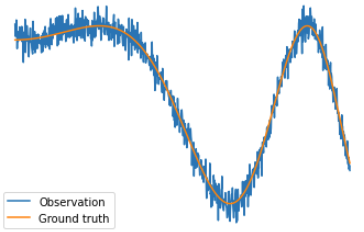Observation and Ground truth

# Creating hidden layers in the network

1. In this section, we will create a feedforward neural network with two hidden layers which contains 20 neurons in each layer. We create this model with a respective weight and bias parameters and finally computing the forward pass of this model. The forward pass is a function which takes input as a parameter and computes the affine function output Y=WX+B, where X is input and Y is output. W is weight, B is a bias which we considered as an unknown parameter θ in the above discussion.

We use tanh as the activation function at each layer in this example. For more information on activation function, please refer to this article here.

# Training and testing the network

A plot below shows the output of the neural network, Just to make a sense of how the output looks before training the neural network.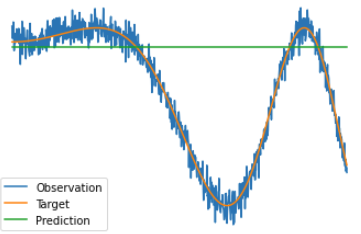Before training NN

As it can be observed, the prediction is just a line, which does not signify the required output.

To train the network, we create a function which calculates the forward pass and loss value using all the trainable variable i.e unknown parameters of the network. In this application, we use Mean square error function which is also called L2 loss. Well, there are many loss functions like L1 loss or Mean absolute error, Hinge loss, L2 loss, categorical loss etc. Choosing the right loss function is important and it depends on the application. Next, we calculate gradient using the calculated loss value and trainable parameters and apply optimization to get the improved value for trainable parameters to fit it perfectly. We use RMSprop (Stochastic gradient descent) optimizer. Again there are many optimizations functions and methods to employ but the usage depends on the application. And finally, the loss value is calculated.

For more details on loss function and Optimizers:

Loss functions

TF Optimizer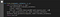Optimization and Loss value.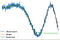Prediction

In this part, the neural network is trained for N-epochs with a specific batch size B and try to minimize the loss function using the optimizer(Gradient Descent). In most cases, the neural network suffers overfitting problems, where the network simply memorizes the training data. This can be observed when the training loss is very low and the test error is very high. This problem can be mitigated by using regularization. I will be explaining the problem of overfitting and the regularization in the next article. Until then see you and happy learning to all.

Deep Learning Enthusiast | Masters Student University of Stuttgart.

## More from Srijayjk

Deep Learning Enthusiast | Masters Student University of Stuttgart.